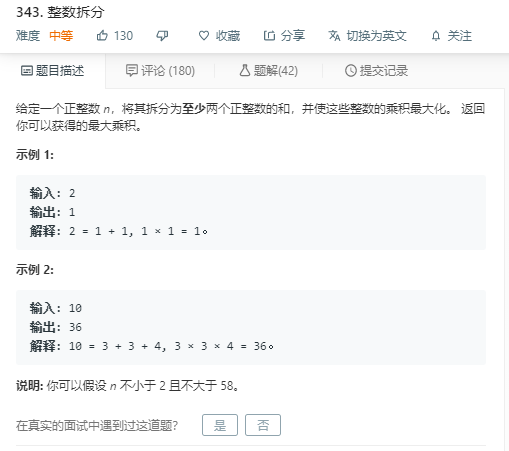### 题目描述#### 我的解法(贪心)

class Solution:
def integerBreak(self, n: int) -> int:
dp =  * 60
dp,dp,dp = 1,2,4
for i in range(5,59):
x = i // 3
y = (i - 3*x) // 2
while(x*3+y*2 != i):
x -= 1
y = (i - 3*x)// 2
dp[i] = 3**x * 2**y
return dp[n]


#### Note:

##### @解题思路：
n拆分方式乘积
21+11不拆分，2>1*1
31+22不拆分，3>1*2
42+24拆分
52+36拆分
63+39拆分
72+2+312拆分

• 结论1：大数字尽可能拆为小因子，通过指数增长获得更大的乘积
• 数字应尽可能拆成尽可能多3的和，但余数为1时 应该把最后1个3+1变为1+1,这就是对我上面给的算法优化的方法。
• 我自己的方法一开始想到的是dp写着写着就变成贪心了 开了个数组 实际上没必要。

#### 细节上更优的贪心解法

class Solution:
def integerBreak(self, n: int) -> int:
if n <= 3 : return n-1
a, b = n // 3, n % 3
if(b==0):return pow(3,a)
if(b==1):return pow(3,a-1)*4
return pow(3,a)*2


#### 动态规划法

class Solution:
def integerBreak(self, n):
dp = [1 for _ in range(n + 1)]
dp = 0
dp = 1
dp = 1
    for i in range(3, n + 1):
dp[i] = max(max(dp[i - 1], i - 1),
2 * max(dp[i - 2], i - 2),
3 * max(dp[i - 3], i - 3))
return dp[n]



#### Note: ##### @解题思路： 动态规划好像都是一开始很难想。。。但是看又很容易看得懂。。。 初始的motivation这道题我也没想明白=。= 积攒经验吧。。。